The vast majority of the nuclides found in nature are stable. If our planet is 4.6 billion years old, the only radioactive isotopes that should remain are members of three classes.

• Isotopes with half-lives of at least 109 years, such as 238U.
• Daughter nuclides produced when long-lived radioactive nuclides decay, such as the234Th (t1/2 = 24.1 days) produced by the-decay of 238U.
• Nuclides such as 14C that are still being synthesized.

One factor that influences the stability of a nuclide is the ratio of neutrons to protons. (Nuclei that contain either too many or too few neutrons are unstable.) Another factor that affects the stability of nuclides can be understood by examining patterns in the numbers of protons and neutrons in stable nuclides.

Half of the elements in the periodic table must have an odd number of protons because atomic numbers that are odd are just as likely to occur as those that are even. In spite of this, about 80% of the stable nuclides have an even number of protons. Very few elements with an odd atomic number have more than one stable isotope. Stable isotopes abound, however, among elements with even atomic numbers. Ten stable isotopes are known for tin (Z = 50), for example. It is also interesting to note that 91% of the stable isotopes of elements with an odd number of protons have an even number of neutrons. These observations suggest that certain combinations of protons and neutrons are particularly stable.

There are magic numbers of electrons. Electron configurations with 2, 10, 18, 36, 54, and 86 electrons are unusually stable. There also seem to be magic numbers of neutrons and protons. Nuclei with 2, 8, 20, 28, 50, 82, or 126 protons or neutrons are unusually stable. This observation explains the anomalously large binding energies observed for 4He, 16O, and 20Ne. In each case, the nuclide has an even number of both protons and neutrons. 20Ne has a magic number of nucleons when both protons and neutrons are counted. 4He and 16O have magic numbers of both protons and neutrons. The resulting stability of the 4He nucleus might explain why so many heavy nuclei undergo-particle decay by ejecting an 4He2+ ion from the nucleus of the atom.

If nuclei tend to be more stable when they have even numbers of protons and neutrons, it isn't surprising that nuclides with an odd number of both protons and neutrons are unstable. 40K is one of only five naturally occurring nuclides that contain both an odd number of protons and an odd number of neutrons. This nuclide simultaneously undergoes the electron capture and positron emission expected for neutron-poor nuclides and the electron emission observed with neutron-rich nuclides.

Only 18 radioactive isotopes with atomic numbers of 80 or less can be found in nature. With the exception of 14C, which is continuously synthesized in the atmosphere, all these elements have lifetimes longer than 109 years. Although these isotopes all undergo radioactive decay, they decay so slowly that reasonable quantities are still present, 4.6 billion years after the planet was formed.

Another 45 natural radioactive isotopes have atomic numbers larger than 80. These nuclides fall into three families. The parent nuclide is 232Th, which undergoes-decay to form 228Ra. The product of this reaction decays by �/i>-emission to form 228Ac, which decays to 228Th, and so on, until the stable 208Pb isotope is formed. This family of radionuclides is called the 4n series, because all its members have a mass number that can be divided by 4.

A second family of radioactive nuclei starts with 238U and decays to form the stable 206Pb isotope. Every member of this series has a mass number that fits the equation 4n + 2. The third family, known as the 4n + 3 series, starts with 235U and decays to 207Pb. A 4n + 1 series once existed, which started with 237Np and decayed to form the only stable isotope of bismuth, 209Bi. The half-life of every member of this series is less than 2 x 106 years, however, so none of the nuclides produced by the decay of neptunium remain in detectable quantities on the earth.In 1934, Irene Curie, the daughter of Pierre and Marie Curie, and her husband, Frederic Joliot, announced the first synthesis of an artificial radioactive isotope. They bombarded a thin piece of aluminum foil with-particles produced by the decay of polonium and found that the aluminum target became radioactive. Chemical analysis showed that the product of this reaction was an isotope of phosphorus.In the next 50 years, more than 2000 other artificial radionuclides were synthesized.

A shorthand notation has been developed for nuclear reactions such as the reaction discovered by Curie and Joliot. The parent (or target) nuclide and the daughter nuclide are separated by parentheses that contain the symbols for the particle that hits the target and the particle or particles released in this reaction.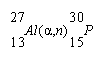The nuclear reactions used to synthesize artificial radionuclides are characterized by enormous activation energies. Three devices are used to overcome these activation energies: linear accelerators, cyclotrons, and nuclear reactors. Linear accelerators or cyclotrons can be used to excite charged particles such as protons, electrons,-particles, or even heavier ions, which are then focused on a stationary target. The following reaction, for example, can be induced by a cyclotron or linear accelerator.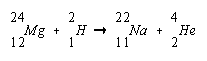Because these reactions involve the capture of a positively charged particles, they usually produce a neutron-poor nuclide.

Artificial radionuclides are also synthesized in nuclear reactors, which are excellent sources of slow-moving, or thermal neutrons. The absorption of a neutron usually results in a neutron-rich nuclide. The following neutron absorption reaction occurs in the cooling systems of nuclear reactors cooled with liquid sodium metal.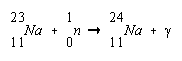In 1940, absorption of thermal neutrons was used to synthesize the first elements with atomic numbers larger than the heaviest naturally occurring element, uranium. The first of these truly artificial elements were neptenium and plutonium, which were synthesized by McMillan and Abelson by irradiating 238U with neutrons to form 239U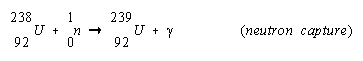which undergoes �/i>--decay to form 239Np and then 239Pu.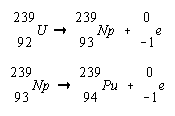Larger bombarding particles were eventually used to produce even heavier transuranium elements.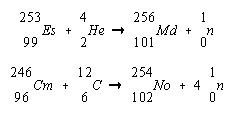The half-lives for-decay and spontaneous fission decrease as the atomic number of the element increases. Element 104, for example, has a half-life for spontaneous fission of 0.3 seconds. Elements therefore become harder to characterize as the atomic number increases. Recent theoretical work has predicted that a magic number of protons might exist at Z = 114. This work suggests that there is an island of stability in the sea of unstable nuclides, as shown in the figure below. If this theory is correct, superheavy elements could be formed if we could find a way to cross the gap between elements Z = 109 through Z = 114.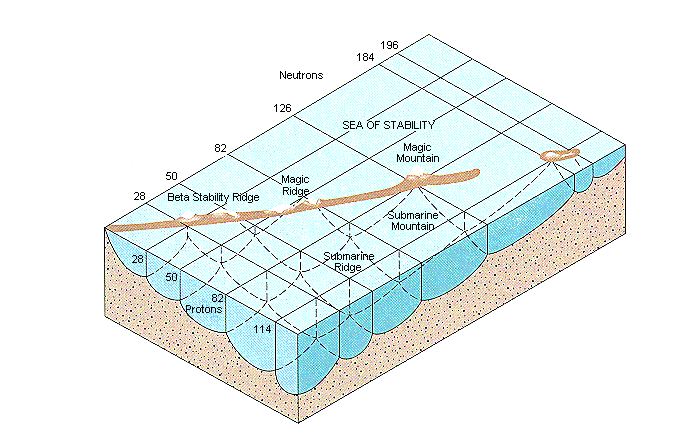There is some debate about the number of neutrons needed to overcome the proton-proton repulsion in a nucleus with 114 protons. The best estimates suggest that at least 184 neutrons, and perhaps as many as 196, would be needed. It is not an easy task to bring together two particles that give both the correct number of total protons and the necessary neutrons to produce a nuclide with a half-life long enough to be detected. If we start with a relatively long-lived parent nuclide, such as 251Cf (t1/2 = 800 y) and bombard this nucleus with a heavy ion, such as 32S, we can envision producing a daughter nuclide with the correct atomic number, but the mass number would be too small by at least 16 amu.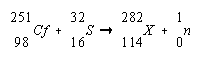Practice Problem 11:Predict the group in the periodic table in which element 114 belongs.  What oxidation states are expected for this element? Click here to check your answer to Practice Problem 11. Click here to see a solution to Practice Problem 11.

An expanded periodic table for elements up to Z = 168 is shown in the figure below. Elements 104 through 112 are transition metals that fill the 6d orbitals. Elements 113 through 120 are main-group elements in which the 7p and 8s orbitals are filled. The next subshell is the 5g atomic orbital, which can hold up to 18 electrons. There is reason to believe that the 5g and 6f orbitals will be filled at the same time. The next 32 elements are therefore grouped into a so-called superactinide series.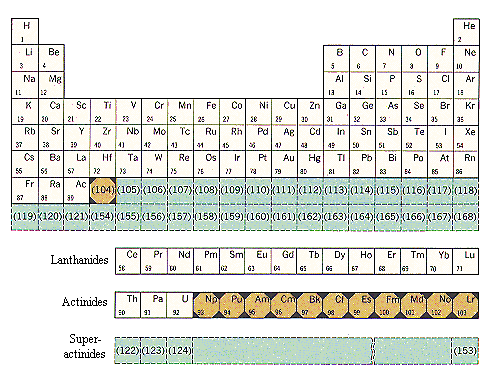An extended version of the periodic table that predicts the positions for all elements up to atomic number 168.

More Detailed Periodic Table